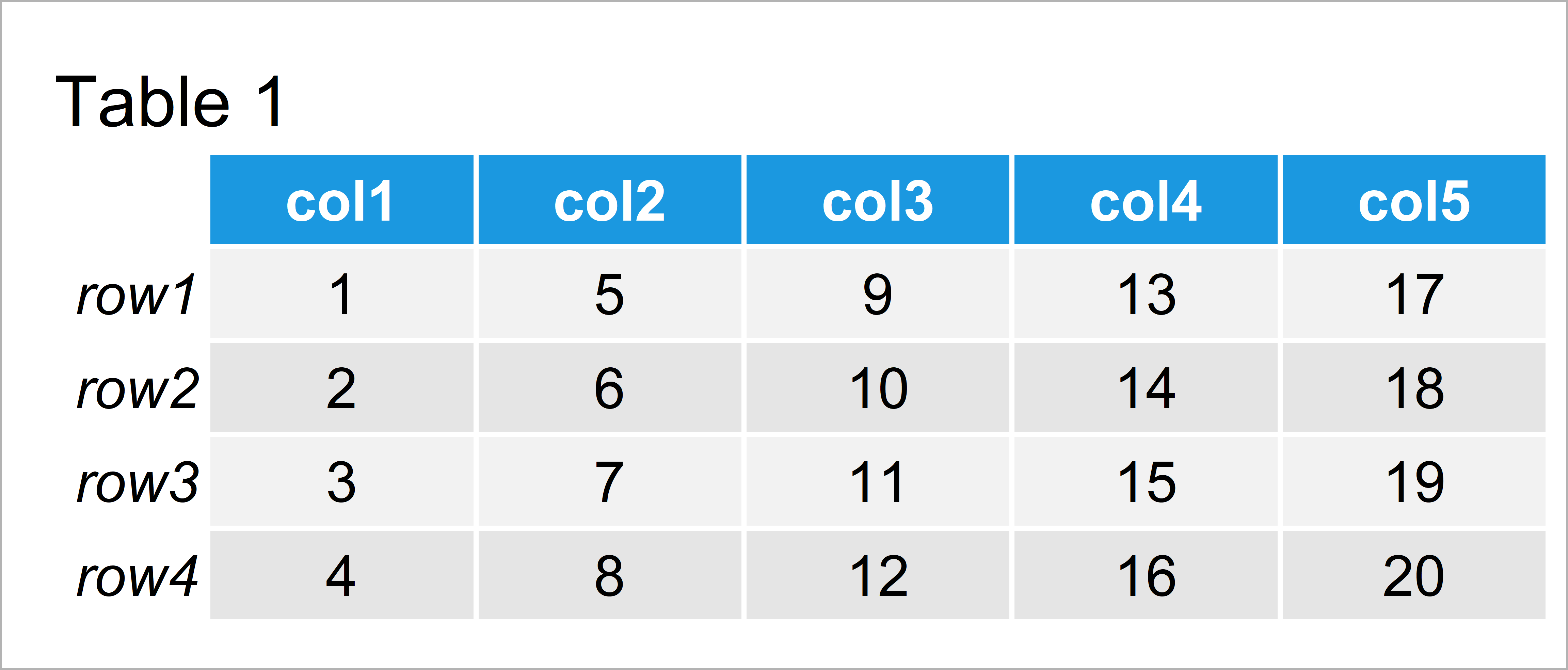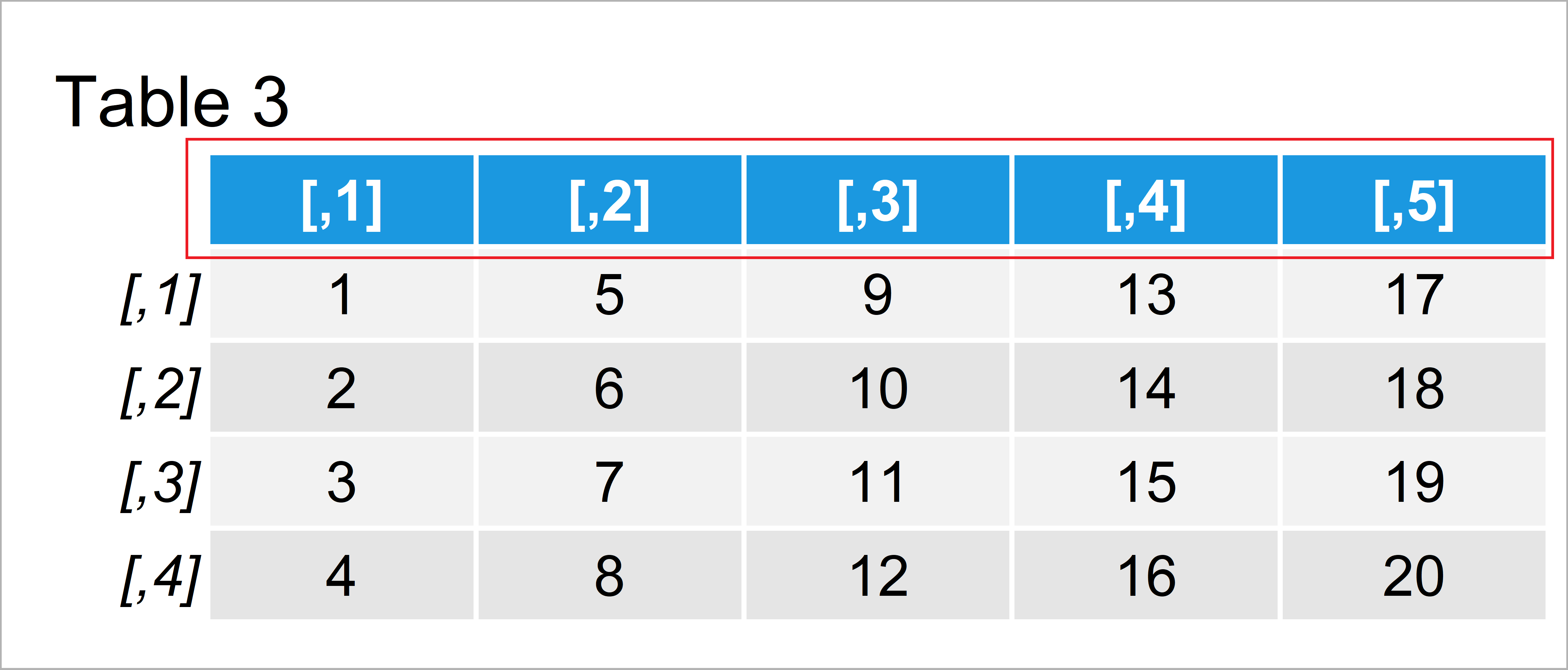# Remove Row & Column Names from Matrix in R (2 Examples)

In this article you’ll learn how to drop all row and column names of a matrix object in R.

Let’s start right away!

## Example Data

We use the following data as basement for this R tutorial:

```mat <- matrix(1:20, ncol = 5)    # Create example matrix
rownames(mat) <- paste0("row", 1:nrow(mat))
colnames(mat) <- paste0("col", 1:ncol(mat))
mat                              # Print example matrix```Table 1 visualizes the output of the RStudio console and shows the structure of our exemplifying matrix: It consists of four rows and five variables.

## Example 1: Remove Row Names from Matrix Using rownames() Function

In this example, I’ll show how to drop row names of a matrix object using the rownames function and NULL.

Have a look at the following R code and its output:

```rownames(mat) <- NULL            # Delete row names
mat                              # Print updated matrix```By running the previous R code we have managed to construct Table 2, i.e. a matrix without row names.

## Example 2: Remove Column Names from Matrix Using colnames() Function

This section shows how to remove variable names from our matrix using the colnames function.

Similar to the previous example, we can assign NULL to our column names to remove all names from our data:

```colnames(mat) <- NULL            # Delete column names
mat                              # Print updated matrix```In Table 3 it is shown that we have created a matrix without column names by running the previous syntax.

## Video & Further Resources

I have recently published a video on my YouTube channel, which shows the R programming syntax of this tutorial. You can find the video below:

Furthermore, you might want to read the related articles of my homepage. I have released numerous articles about matrices already:

This article has illustrated how to unname matrix objects in the R programming language. In case you have additional questions, don’t hesitate to let me know in the comments section.

Subscribe to the Statistics Globe Newsletter